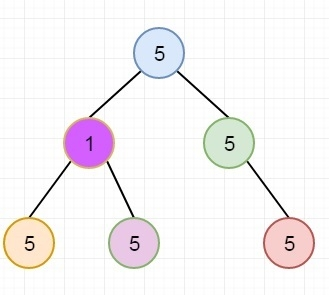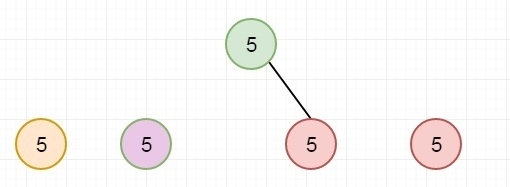# Find Count of Single Valued Subtrees in C++

Suppose we have a binary tree. Our task is to count single valued subtrees in the given tree. A single valued subtree is a subtree, where all nodes of that tree is containing same value. Suppose a tree is like below −There are four Single value subtrees. These are like below −We can solve this using bottom up manner. For every subtree visited, return true, if the subtree, rooted under it is single valued, and increase the counter by 1. Here count is the reference parameter for recursive call. And use the returned value to find out if left and right subtrees are single valued or not.

## Example

Live Demo

#include <iostream>
using namespace std;
class Node {
public:
int data;
Node* left, *right;
};
Node* getNode(int data) {
Node* newNode = new Node;
newNode->data = data;
newNode->left = newNode->right = NULL;
return newNode;
}
bool countSingleValuedSubtree(Node* root, int &count) {
if (root == NULL)
return true;
bool left = countSingleValuedSubtree(root->left, count);
bool right = countSingleValuedSubtree(root->right, count);
if (left == false || right == false)
return false;
if (root->left && root->data != root->left->data)
return false;
if (root->right && root->data != root->right->data)
return false;
count++;
return true;
}
int countSingleValSubtree(Node* root) {
int count = 0;
countSingleValuedSubtree(root, count);
return count;
}
int main() {
Node* root = getNode(5);
root->left = getNode(1);
root->right = getNode(5);
root->left->left = getNode(5);
root->left->right = getNode(5);
root->right->right = getNode(5);
cout << "Count of Single Valued Subtrees is: " << countSingleValSubtree(root);
}

## Output

Count of Single Valued Subtrees is: 4